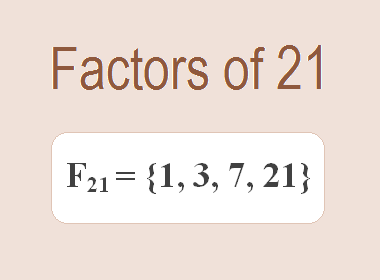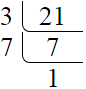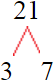# Factors of 21The factors of 21 are 1, 3, 7, and 21 i.e. F21 = {1, 3, 7, 21}. The factors of 21 are those numbers that can divide 21 without leaving a remainder.

We can check if these numbers are factors of 21 by dividing 21 by each of them. If the result is a whole number, then the number is a factor of 21. Let's do this for each of the numbers listed above:

·        1 is a factor of 21 because 21 divided by 1 is 21.

·        3 is a factor of 21 because 21 divided by 3 is 7.

·        7 is a factor of 21 because 21 divided by 7 is 3.

·        21 is a factor of 21 because 21 divided by 21 is 1.

## How to Find Factors of 21?

1 and the number itself are the factors of every number. So, 1 and 21 are two factors of 21. To find the other factors of 21, we can start by dividing 21 by the numbers between 1 and 21. If we divide 21 by 2, we get a remainder of 1. Therefore, 2 is not a factor of 21. If we divide 21 by 3, we get a remainder of 0. Therefore, 3 is a factor of 21.

Next, we can check if 4 is a factor of 21. If we divide 21 by 4, we get a remainder of 1. Therefore, 4 is not a factor of 21. We can continue this process for all the possible factors of 21.

Through this process, we can find that the factors of 21 are 1, 3, 7, and 21. These are the only numbers that can divide 21 without leaving a remainder.********************

********************

## Properties of the Factors of 21

The factors of 21 have some interesting properties. One of the properties is that the sum of the factors of 21 is equal to 32. We can see this by adding all the factors of 21 together:

1 + 3 + 7 + 21 = 32

Another property of the factors of 21 is that they are all odd numbers. This is because 21 is an odd number, and any even number cannot divide an odd number without leaving a remainder.

Another property of the factors of 21 is that they are all prime numbers except 1 and 21.

## Applications of the Factors of 21

The factors of 21 have several applications in mathematics. One of the applications is in finding the highest common factor (HCF) of two or more numbers. The HCF is the largest factor that two or more numbers have in common. For example, to find the HCF of 21 and 28, we need to find the factors of both numbers and identify the largest factor they have in common. The factors of 21 are 1, 3, 7, and 21. The factors of 28 are 1, 2, 4, 7, 14, and 28. The largest factor that they have in common is 7. Therefore, the HCF of 21 and 28 is 7.

Another application of the factors of 21 is in prime factorization. Prime factorization is the process of expressing a number as the product of its prime factors. The prime factors of 21 are 3 and 7 since these are the only prime numbers that can divide 21 without leaving a remainder. Therefore, we can express 21 as:

21 = 3 × 7

We can do prime factorization by division and factor tree method also. Here is the prime factorization of 21 by division method,21 = 3 × 7

Here is the prime factorization of 21 by the factor tree method,21 = 3 × 7

## Conclusion

The factors of 21 are the numbers that can divide 21 without leaving a remainder. The factors of 21 are 1, 3, 7, and 21. The factors of 21 have some interesting properties, such as being odd numbers and having a sum of 32. The factors of 21 have several applications in mathematics, such as finding the highest common factor and prime factorization.